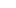# Solutions

Get detailed explanations to advanced GMAT questions.

### QuestionIn the figure above, what is the length of segment PQ ?

I. S is the midpoint of segment RT

II. Segment PT has length 10 and segment QT has length 6.

Option A:

Statement (1) ALONE is sufficient, but statement (2) alone is not sufficient to answer the question asked.

Option B:

Statement (2) ALONE is sufficient, but statement (1) alone is not sufficient to answer the question asked.

Option C:

BOTH statements (1) and (2) TOGETHER are sufficient to answer the question asked, but NEITHER statement ALONE is sufficient to answer the question asked.

Option D:

Option E:

Statements (1) and (2) TOGETHER are NOT sufficient to answer the question asked, and additional data specific to the problem are needed.

Easy

### Solution

Option B is the correct answer.

### Option Analysis

Length of line segment PQ?

Statement I is insufficient: From this, we only infer that RS = ST. Nothing about PQ. So not sufficient.

Statement II is sufficient:

If PT = 10 and QT = 6

Then PQ = 4

Sufficient.